# ARRAYS IN JAVA

Arrays In Java | Core Java Tutorial | Minigranth

# Arrays in Java : Introduction

• Arrays in java are considered as objects. Arrays are the collection of homogeneous (same type) elements referred to by a single name.
• Arrays elements can be accessed randomly using index. In Java array index starts from 0.
• Arrays in java are of two types:
• One- Dimensional Arrays
• Multi- Dimensional Arrays
1. ### Arrays in Java : One Dimensional Array

• 1-D array is the collection of homogeneous elements. We can represent 1-D array as a single row of elements.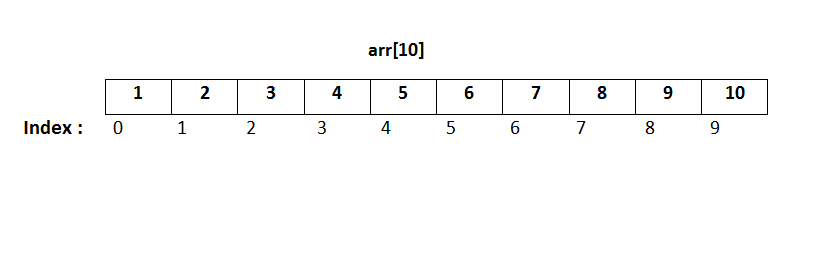Arrays In Java : One-Dimension Array
• The above diagram represents an integer array arr of size 10 and index going from 0 to 9.
• If size of array is ‘n’ the index goes from 0 to n-1.

Declaring Array
 type [ ] array_name ;  or type array_name[ ] ;

• Type is the data type of the array elements. It will tell what type of data array will hold.
• [ ] tells the compiler that the variable is an array variable. For example : int [ ]  arr ;
• Declaring an array does not mean that array exist in memory. Declaring an array will set arr to to null.
• We have to allocate memory to array using new keyword.

Syntax
 array_name = new type[size] ;

• "size" tells the number of elements array can hold. Initial allocation of memory to an array initializes all array elements to 0.
• We can assign an array element with a new value by using array_name and index.
• For example
arr = new int;   // will allocate memory to an array of size 5
arr = 10;   // will assign the first element of array to 10
arr = 40;   //  will assign the fourth element of array to 40

 Note : 1) In Java array are dynamically allocated, i.e. memory to an array is allocated at run time. 2) We can traverse an array using a loop ( mainly for-each loop). 3) We can also declare and allocate memory to an array with a single statement by combining both declaration and allocation statement. For example :  int[ ] arr = new int  ;

Example
• Let us take an example to demonstrate arrays in java :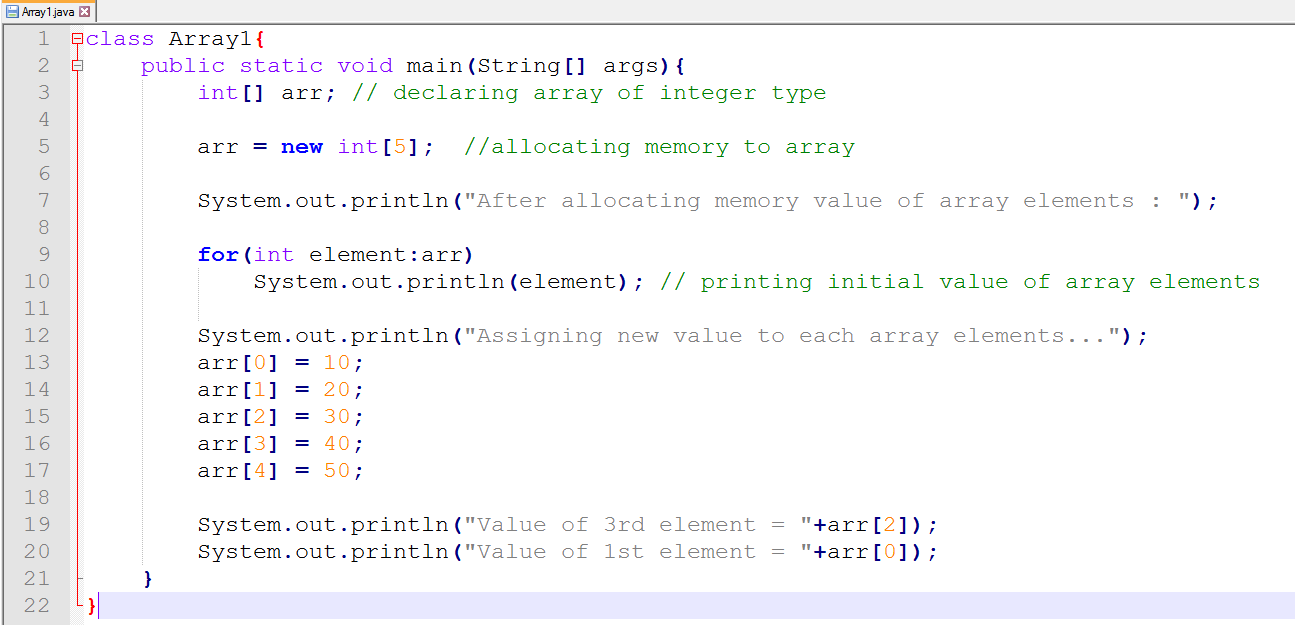Arrays In Java : Example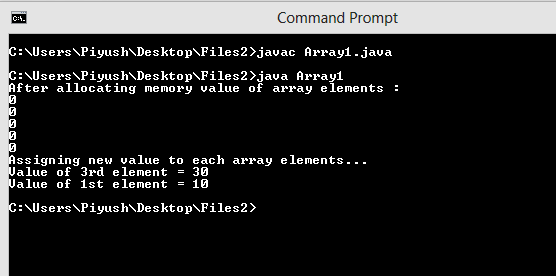Arrays In Java : Output

Initializing an Array While Declaring it
• Array can be initialized while they are declared. We don’t need new keyword to allocate memory. It is allocated automatically.

Syntax
 type[ ] array_name = { List of elements separated by comma};

Example

char[ ] alpha = {‘a’, ‘b’, ‘c’, ‘d’, ‘e’, ‘f’};

• The above statement will declare and initialize a character array alpha at the same time. There is no need of new keyword then. Comma (,) separates the different array elements.
• Let us take an example to find mean of heights of 10 persons.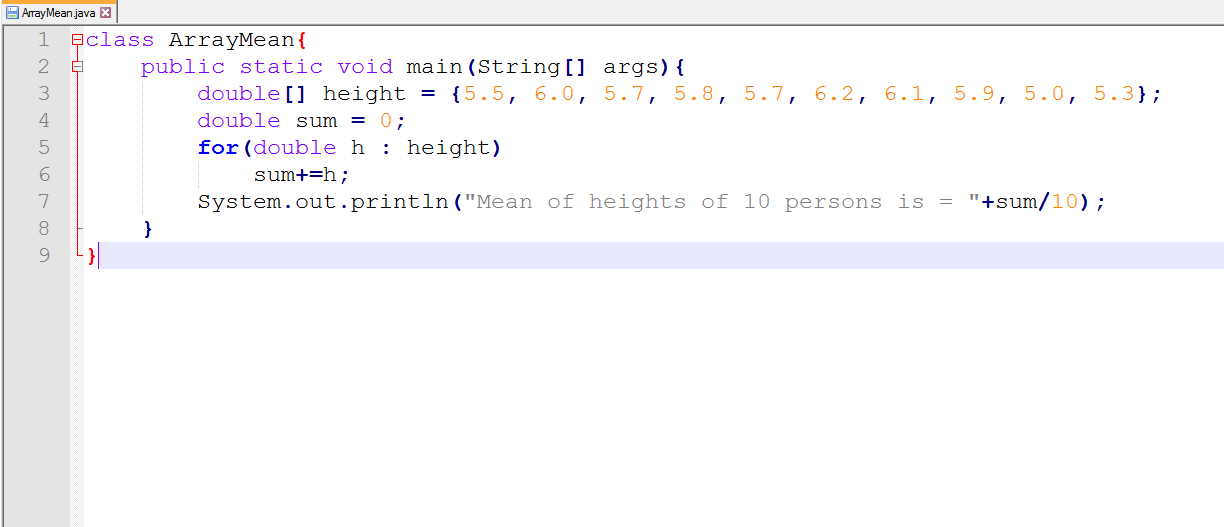1-D Array : Example1-D Array : Output

2. ### Arrays In Java : Multi- Dimensional Arrays

• Array can be multidimensional.
• Conceptually multidimensional arrays are represented as having multiple rows and columns.
• Multi- dimensional array can be 2-dimensional, 3-dimensional up-to n-dimensional.
• Multi-dimensional arrays are simply array within an array. If we talk about 2-D array then they are array within array i.e. array(array) .
• If we talk about 3-D array they are array within array within array i.e. array(array(array)) .
• The simplest multidimensional array is 2-D array. Conceptually they can be represented as having more than one row ( matrix like structure).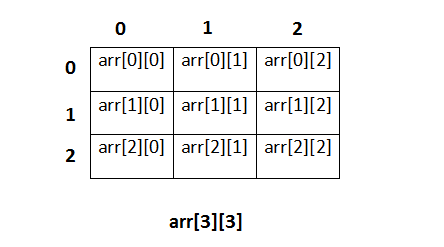Multidimensional Array : Structure

• Above figure is the conceptual representation of 2-D array having 3 rows and 3 columns.
• If we write arr, then it represents element of 2nd row and 3rd Here 1 is the row index and 2 is the column index.

Declaring Multi-Dimensional Array
• To declare 2-D array we specify two brackets [ ] [ ]. n dimension means n brackets. For example:
• int[ ] [ ] arr ;// declaring 2-D array
• int [ ] [ ] [ ] arr1 ;   // declaring 3-D array

Allocation Memory to Multi- Dimensional Array
• It is done using new keyword, as we did in 1-D array. But here also we specify n [ ] brackets for n dimension. For example:
• arr = new int ;  // 2-D array of 4 rows and 5 columns

Allocating While Declaring
•  An example could be , char[ ] [ ] mat = new char ; // Declares and allocates memory

•  Note : We can use loop to traverse multidimensional array.

Example
• Let us take an example to demonstrate 2-D arrays.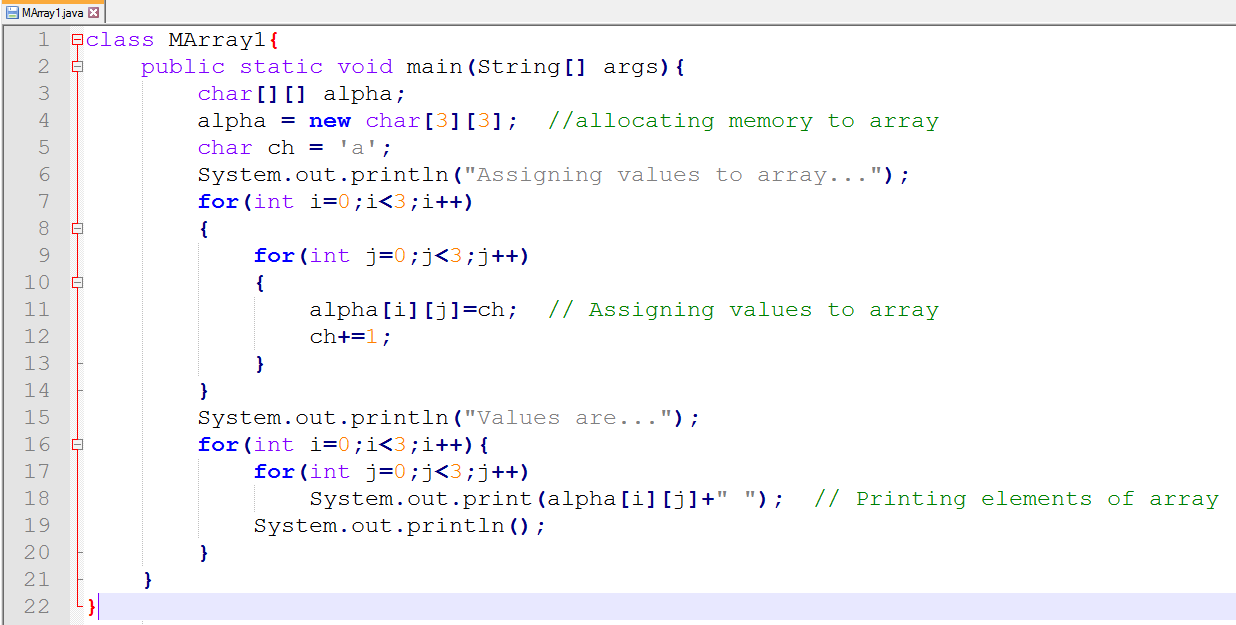Multi-Dimensional Array : ExampleMulti-Dimensional Array : Output

Initializing 2-D Array While Declaring
• We can also declare and initialize 2-D array at the same time as we did in 1-D array.
• In case of 2-D array each set of rows will have their own set of curly braces. For example
• int[ ][ ] arr ={ {1,2,3}, {4,5,6}, {7,8,9} } ;
• The above statement will create a 2-D array of size 3 X 3.
• Let us take an example where we will add two matrices of size 2 X 3.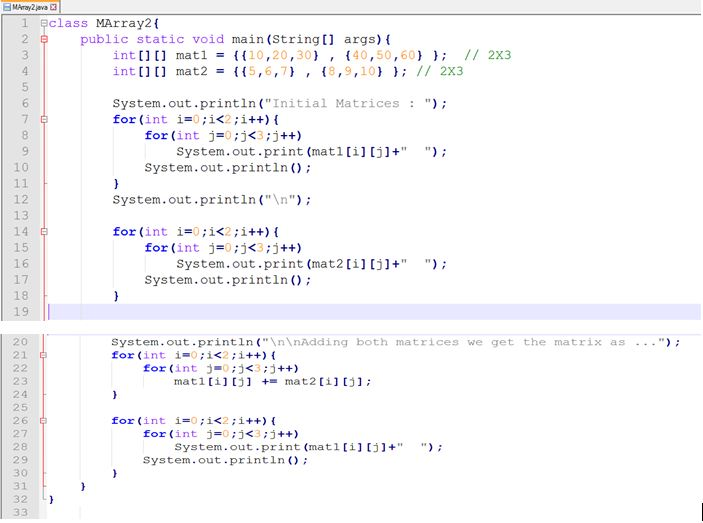Multidimensional Array : Example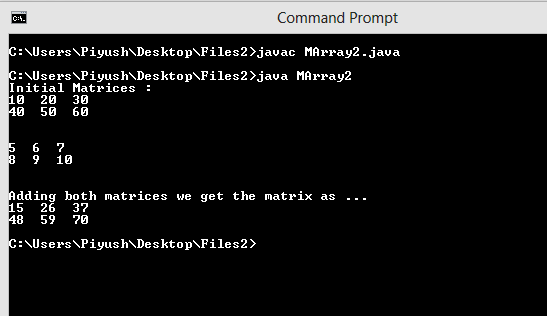Multidimensional Array : Output